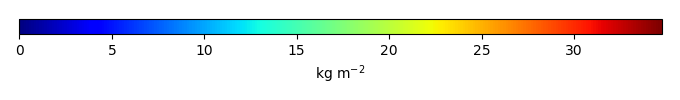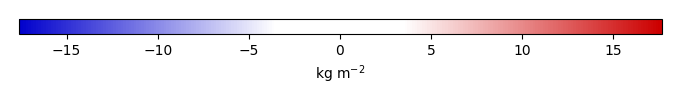# Mean State

Period Mean (original grids) [Pg]
Model Period Mean (intersection) [Pg]
Model Period Mean (complement) [Pg]
Benchmark Period Mean (intersection) [Pg]
Benchmark Period Mean (complement) [Pg]
Bias [kg m-2]
Bias Score 
Spatial Distribution Score 
Overall Score 
Benchmark [-] 64.0
CRUNCEPv7 [-] 42.9 43.1 0.424 63.9 0.0663 -5.75 0.67 0.67 0.67
GSWP3v1 [-] 38.4 38.4 0.429 63.9 0.0663 -7.15 0.63 0.58 0.61
WATCH [-] 39.0 39.4 0.457 63.9 0.0663 -6.86 0.64 0.55 0.59
Period Mean (original grids) [Pg]
Model Period Mean (intersection) [Pg]
Model Period Mean (complement) [Pg]
Benchmark Period Mean (intersection) [Pg]
Benchmark Period Mean (complement) [Pg]
Bias [kg m-2]
Bias Score 
Spatial Distribution Score 
Overall Score 
Benchmark [-] 3.34
CRUNCEPv7 [-] 3.63 2.48 0.742 3.34 0.00700 -0.242 0.53 0.58 0.56
GSWP3v1 [-] 3.30 2.21 0.738 3.34 0.00700 -0.331 0.50 0.57 0.53
WATCH [-] 6.16 4.63 0.862 3.34 0.00700 0.480 0.52 0.80 0.66
Period Mean (original grids) [Pg]
Model Period Mean (intersection) [Pg]
Model Period Mean (complement) [Pg]
Benchmark Period Mean (intersection) [Pg]
Benchmark Period Mean (complement) [Pg]
Bias [kg m-2]
Bias Score 
Spatial Distribution Score 
Overall Score 
Benchmark [-] 14.1
CRUNCEPv7 [-] 17.0 15.6 1.11 14.1 0.0194 0.409 0.56 0.83 0.70
GSWP3v1 [-] 12.3 11.1 0.996 14.1 0.0194 -0.223 0.55 0.79 0.67
WATCH [-] 20.7 19.2 1.13 14.1 0.0194 0.926 0.54 0.84 0.69
Period Mean (original grids) [Pg]
Model Period Mean (intersection) [Pg]
Model Period Mean (complement) [Pg]
Benchmark Period Mean (intersection) [Pg]
Benchmark Period Mean (complement) [Pg]
Bias [kg m-2]
Bias Score 
Spatial Distribution Score 
Overall Score 
Benchmark [-] 1.22
CRUNCEPv7 [-] 5.09 4.27 0.836 1.22 0.00453 1.36 0.37 0.45 0.41
GSWP3v1 [-] 4.18 3.37 0.817 1.22 0.00453 0.991 0.47 0.50 0.49
WATCH [-] 5.42 4.62 0.834 1.22 0.00453 1.50 0.35 0.64 0.49
Period Mean (original grids) [Pg]
Model Period Mean (intersection) [Pg]
Model Period Mean (complement) [Pg]
Benchmark Period Mean (intersection) [Pg]
Benchmark Period Mean (complement) [Pg]
Bias [kg m-2]
Bias Score 
Spatial Distribution Score 
Overall Score 
Benchmark [-] 47.3
CRUNCEPv7 [-] 49.6 47.1 2.28 45.9 1.37 5.89 0.71 0.64 0.68
GSWP3v1 [-] 40.1 38.1 1.81 45.9 1.37 2.33 0.68 0.51 0.60
WATCH [-] 39.0 36.9 1.88 45.9 1.37 1.96 0.66 0.43 0.54
Period Mean (original grids) [Pg]
Model Period Mean (intersection) [Pg]
Model Period Mean (complement) [Pg]
Benchmark Period Mean (intersection) [Pg]
Benchmark Period Mean (complement) [Pg]
Bias [kg m-2]
Bias Score 
Spatial Distribution Score 
Overall Score 
Benchmark [-] 41.6
CRUNCEPv7 [-] 73.2 71.5 0.536 41.6 0.0303 3.57 0.49 0.67 0.58
GSWP3v1 [-] 52.3 51.1 0.450 41.6 0.0303 1.23 0.55 0.74 0.65
WATCH [-] 50.2 49.0 0.389 41.6 0.0303 0.955 0.71 0.94 0.82
Period Mean (original grids) [Pg]
Model Period Mean (intersection) [Pg]
Model Period Mean (complement) [Pg]
Benchmark Period Mean (intersection) [Pg]
Benchmark Period Mean (complement) [Pg]
Bias [kg m-2]
Bias Score 
Spatial Distribution Score 
Overall Score 
Benchmark [-] 15.6
CRUNCEPv7 [-] 36.3 33.8 3.32 15.6 0.0349 2.50 0.45 0.59 0.52
GSWP3v1 [-] 31.8 29.6 2.96 15.6 0.0349 1.93 0.51 0.68 0.59
WATCH [-] 33.4 30.3 3.72 15.6 0.0349 2.00 0.54 0.76 0.65
Period Mean (original grids) [Pg]
Model Period Mean (intersection) [Pg]
Model Period Mean (complement) [Pg]
Benchmark Period Mean (intersection) [Pg]
Benchmark Period Mean (complement) [Pg]
Bias [kg m-2]
Bias Score 
Spatial Distribution Score 
Overall Score 
Benchmark [-] 14.8
CRUNCEPv7 [-] 25.5 23.4 1.50 14.6 0.136 2.67 0.53 0.69 0.61
GSWP3v1 [-] 24.9 22.6 1.43 14.6 0.136 2.37 0.53 0.73 0.63
WATCH [-] 25.6 23.4 1.53 14.6 0.136 2.50 0.55 0.77 0.66
Period Mean (original grids) [Pg]
Model Period Mean (intersection) [Pg]
Model Period Mean (complement) [Pg]
Benchmark Period Mean (intersection) [Pg]
Benchmark Period Mean (complement) [Pg]
Bias [kg m-2]
Bias Score 
Spatial Distribution Score 
Overall Score 
Benchmark [-] 1.78
CRUNCEPv7 [-] 8.91 7.07 2.32 1.78 0.00 2.69 0.31 0.54 0.42
GSWP3v1 [-] 6.90 5.31 1.98 1.78 0.00 1.79 0.40 0.58 0.49
WATCH [-] 5.09 4.34 1.01 1.78 0.00 1.29 0.53 0.86 0.70
Period Mean (original grids) [Pg]
Model Period Mean (intersection) [Pg]
Model Period Mean (complement) [Pg]
Benchmark Period Mean (intersection) [Pg]
Benchmark Period Mean (complement) [Pg]
Bias [kg m-2]
Bias Score 
Spatial Distribution Score 
Overall Score 
Benchmark [-] 15.3
CRUNCEPv7 [-] 11.8 9.77 1.81 14.8 0.505 -0.0658 0.55 0.80 0.67
GSWP3v1 [-] 11.3 9.25 1.86 14.8 0.505 -0.174 0.55 0.81 0.68
WATCH [-] 12.1 9.98 1.90 14.8 0.505 -0.0371 0.56 0.82 0.69
Period Mean (original grids) [Pg]
Model Period Mean (intersection) [Pg]
Model Period Mean (complement) [Pg]
Benchmark Period Mean (intersection) [Pg]
Benchmark Period Mean (complement) [Pg]
Bias [kg m-2]
Bias Score 
Spatial Distribution Score 
Overall Score 
Benchmark [-] 13.1
CRUNCEPv7 [-] 26.8 25.2 1.18 13.1 0.0236 3.21 0.50 0.59 0.55
GSWP3v1 [-] 18.2 17.0 0.899 13.1 0.0236 1.50 0.50 0.69 0.60
WATCH [-] 13.4 12.3 0.812 13.1 0.0236 0.547 0.54 0.80 0.67
Period Mean (original grids) [Pg]
Model Period Mean (intersection) [Pg]
Model Period Mean (complement) [Pg]
Benchmark Period Mean (intersection) [Pg]
Benchmark Period Mean (complement) [Pg]
Bias [kg m-2]
Bias Score 
Spatial Distribution Score 
Overall Score 
Benchmark [-] 455.
CRUNCEPv7 [-] 590. 564. 26.5 449. 5.38 1.95 0.65 0.89 0.77
GSWP3v1 [-] 487. 464. 23.3 449. 5.38 0.776 0.64 0.86 0.75
WATCH [-] 507. 484. 23.4 449. 5.38 0.972 0.67 0.87 0.77
Period Mean (original grids) [Pg]
Model Period Mean (intersection) [Pg]
Model Period Mean (complement) [Pg]
Benchmark Period Mean (intersection) [Pg]
Benchmark Period Mean (complement) [Pg]
Bias [kg m-2]
Bias Score 
Spatial Distribution Score 
Overall Score 
Benchmark [-] 0.854
CRUNCEPv7 [-] 1.62 0.656 0.960 0.849 0.00531 0.00552 0.48 0.64 0.56
GSWP3v1 [-] 1.51 0.664 0.856 0.849 0.00531 0.0462 0.48 0.72 0.60
WATCH [-] 2.22 1.14 1.06 0.849 0.00531 0.389 0.51 0.76 0.64
Period Mean (original grids) [Pg]
Model Period Mean (intersection) [Pg]
Model Period Mean (complement) [Pg]
Benchmark Period Mean (intersection) [Pg]
Benchmark Period Mean (complement) [Pg]
Bias [kg m-2]
Bias Score 
Spatial Distribution Score 
Overall Score 
Benchmark [-] 2.82
CRUNCEPv7 [-] 16.4 13.6 2.63 2.82 0.00299 3.44 0.27 0.31 0.29
GSWP3v1 [-] 14.5 12.3 2.08 2.82 0.00299 3.01 0.27 0.35 0.31
WATCH [-] 11.4 9.42 1.85 2.82 0.00299 2.11 0.36 0.48 0.42
Period Mean (original grids) [Pg]
Model Period Mean (intersection) [Pg]
Model Period Mean (complement) [Pg]
Benchmark Period Mean (intersection) [Pg]
Benchmark Period Mean (complement) [Pg]
Bias [kg m-2]
Bias Score 
Spatial Distribution Score 
Overall Score 
Benchmark [-] 4.95
CRUNCEPv7 [-] 12.0 10.8 0.884 4.88 0.0615 3.93 0.38 0.42 0.40
GSWP3v1 [-] 10.2 9.16 0.789 4.88 0.0615 3.11 0.45 0.52 0.48
WATCH [-] 10.8 9.81 0.800 4.88 0.0615 3.42 0.44 0.54 0.49
Period Mean (original grids) [Pg]
Model Period Mean (intersection) [Pg]
Model Period Mean (complement) [Pg]
Benchmark Period Mean (intersection) [Pg]
Benchmark Period Mean (complement) [Pg]
Bias [kg m-2]
Bias Score 
Spatial Distribution Score 
Overall Score 
Benchmark [-] 13.0
CRUNCEPv7 [-] 17.6 17.1 0.407 13.0 0.00854 1.40 0.64 0.89 0.76
GSWP3v1 [-] 11.8 11.4 0.332 13.0 0.00854 -0.100 0.65 0.87 0.76
WATCH [-] 14.0 13.6 0.317 13.0 0.00854 0.422 0.69 0.89 0.79
Period Mean (original grids) [Pg]
Model Period Mean (intersection) [Pg]
Model Period Mean (complement) [Pg]
Benchmark Period Mean (intersection) [Pg]
Benchmark Period Mean (complement) [Pg]
Bias [kg m-2]
Bias Score 
Spatial Distribution Score 
Overall Score 
Benchmark [-] 21.4
CRUNCEPv7 [-] 57.0 56.6 0.599 21.3 0.128 7.37 0.37 0.70 0.54
GSWP3v1 [-] 43.8 43.5 0.471 21.3 0.128 4.72 0.47 0.79 0.63
WATCH [-] 39.2 38.9 0.429 21.3 0.128 3.78 0.54 0.88 0.71
Period Mean (original grids) [Pg]
Model Period Mean (intersection) [Pg]
Model Period Mean (complement) [Pg]
Benchmark Period Mean (intersection) [Pg]
Benchmark Period Mean (complement) [Pg]
Bias [kg m-2]
Bias Score 
Spatial Distribution Score 
Overall Score 
Benchmark [-] 9.57
CRUNCEPv7 [-] 3.52 3.30 0.245 9.53 0.0397 -1.79 0.50 0.65 0.57
GSWP3v1 [-] 3.09 2.90 0.233 9.53 0.0397 -1.92 0.49 0.49 0.49
WATCH [-] 3.38 3.16 0.245 9.53 0.0397 -1.85 0.49 0.52 0.50
Period Mean (original grids) [Pg]
Model Period Mean (intersection) [Pg]
Model Period Mean (complement) [Pg]
Benchmark Period Mean (intersection) [Pg]
Benchmark Period Mean (complement) [Pg]
Bias [kg m-2]
Bias Score 
Spatial Distribution Score 
Overall Score 
Benchmark [-] 151.
CRUNCEPv7 [-] 152. 151. 0.651 151. 0.0403 0.381 0.80 0.87 0.84
GSWP3v1 [-] 129. 128. 0.570 151. 0.0403 -2.41 0.76 0.78 0.77
WATCH [-] 145. 144. 0.555 151. 0.0403 -0.517 0.81 0.86 0.84
Period Mean (original grids) [Pg]
Model Period Mean (intersection) [Pg]
Model Period Mean (complement) [Pg]
Benchmark Period Mean (intersection) [Pg]
Benchmark Period Mean (complement) [Pg]
Bias [kg m-2]
Bias Score 
Spatial Distribution Score 
Overall Score 
Benchmark [-] 10.7
CRUNCEPv7 [-] 21.4 21.3 0.571 10.7 0.0170 1.85 0.51 0.81 0.66
GSWP3v1 [-] 21.5 21.4 0.512 10.7 0.0170 1.83 0.52 0.81 0.66
WATCH [-] 23.6 23.5 0.530 10.7 0.0170 2.14 0.52 0.83 0.68
Period Mean (original grids) [Pg]
Model Period Mean (intersection) [Pg]
Model Period Mean (complement) [Pg]
Benchmark Period Mean (intersection) [Pg]
Benchmark Period Mean (complement) [Pg]
Bias [kg m-2]
Bias Score 
Spatial Distribution Score 
Overall Score 
Benchmark [-] 4.41
CRUNCEPv7 [-] 5.25 4.84 0.454 4.31 0.102 1.14 0.59 0.77 0.68
GSWP3v1 [-] 6.25 5.78 0.493 4.31 0.102 1.41 0.59 0.80 0.70
WATCH [-] 5.95 5.52 0.476 4.31 0.102 1.20 0.62 0.84 0.73

# Temporally integrated period mean

BENCHMARK MEANMODEL MEANBIASBIAS SCORESPATIAL TAYLOR DIAGRAMMODEL COLORS# Spatially integrated regional mean

MODEL COLORS# All Models

BenchmarkCRUNCEPv7GSWP3v1WATCH# Data Information

history: Mon Mar 21 09:05:59 2016: ncatted -a units,biomass,o,c,kg m-2 ./from_MQs_site/DATA/biomass/GEOCARBON/derived/biomass_0.5x0.5.nc tmp.nc